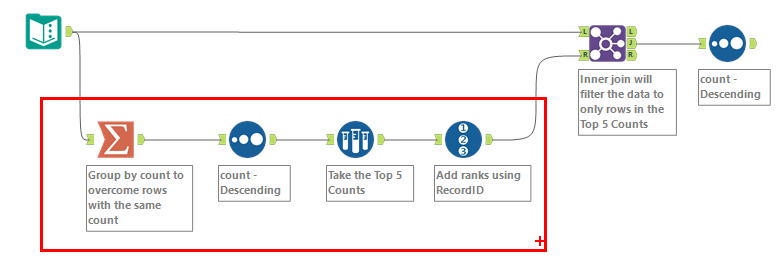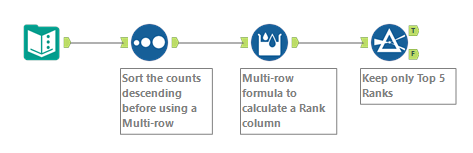## Ranking (Dense) in Alteryx

by Joselito Bondoc

During an Alteryx Challenge (challenge 9), I found that creating a dense ranking in Alteryx isn't straightforward. So I will be showing two methods: using a lookup table and using the Multi-row formula tool.

What is dense ranking?

• values that are equal are given the same ranking number
• the next following value will be given the immediate ranking number, no ranking numbers are skipped
• i.e. 1, 1, 2, 3, 4, 4, 5

## Alteryx Challenge 9

In figure a. we can see that multiple names have the same count, so we would like to give these players the same count.

### Method 1: Creating a lookup tableFigure b. The flow section enclosed above creates our lookup table.
• Summarise: We are grouping by the count field to obtain a single row for each unique count value.
• Sort: Sorting the count field from highest to lowest (descending)
• Sample: This tool can be skipped but it was required for the challenge.
• RecordID: Adds a rank field, assigning a ranking number for each unique count value
The result of these steps is shown in the figure below:

The created lookup table can then be used to join (figure b.) back to the starting dataset in order to assign ranks. The result of this (after using another sort tool) is shown below:

### Method 2: Using the Multi-row formulaFigure e. Workflow to create the same ranking output using the multi-row formula
• Sort: Similar to the first method, the count field has to be sorted in descending order
• Multi-row formula: Creates the dense ranking column (more on this below)
• Filter: Requirement for the challenge. Can be skipped

### What is the Multi-row formula doing?

• Creating a new field called 'Rank'
• Values for Rows that don't exist is set to 0 or Empty

The expression will compare the current count value to the previous count value, if they are equal then the rank of the current value is the same as the previous. Otherwise, the next ranking number will be assigned.

Settings the value for rows that don't exist as 0 or Empty is important because for the first row, a previous count value doesn't exist so it is set to 0 and the IF statement returns FALSE. Therefore, the first row is given the previous row's rank (which doesn't exist so it is set to 0) + 1, i.e. 0 + 1 = 1. Following this, the subsequent rankings are then populated row by row, which gives the same output as figure d.

Thumbnail image from Joshua Golde on Unsplash.Joselito Bondoc

Tue 15 Jun 2021

Thu 03 Jun 2021

Wed 02 Jun 2021